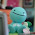## Thursday, January 13, 2011

### Final Percent Post

What is a percent?

Percent is a number out of 100.
For example 75% is 75 out of 100.
Percents can be expressed by using decimals and fractions.
For example 75% is also 0.75 and 75/100.

Key Points for this Chapter

4.1 Representing Percents

To represent a percent you can shade squares on a grid out of 100 squares called a hundred grid

To represent a percent greater than 100% shade more than one grid

To represent a fractional percent between 0% and 1% shade part of one square

4.2 Fractions, Decimals and Percents

Fractions, decimals and percents can be used to represent numbers in various situations

Percents can be written as fractions and decimals

4.3 Percent of a Number

You can use mental math strategies like halving, doubling and dividing by 10 to find the percents of some numbers

To calculate the percent of a number, write the percent as a decimal and then multiply by the number

4.4 Combining Percents

Percents can be combined by adding to solve problems

To calculate the increase in a number: Add the combined percent amount to the original number, or multiply the original number by a single percent greater than 100

Here is my video explaining percents

Here's a video using a percent question to show how to find a percent of a number

And finally this is the link to my percent scribe post.

Hope you liked my video and post.

#### 1 comment:

1.Great post Nils! You had colour, you had a video, you followed the instructions. Great Job! One thing that I wish you can do is add the things to the left because it was sort of dufficult for me to read when everything was not in the right place, other than that great job!